# A note on the Aiello–Subbarao conjecture on addition chains

Hatem M. Bahig
Notes on Number Theory and Discrete Mathematics
Print ISSN 1310–5132, Online ISSN 2367–8275
Volume 28, 2022, Number 2, Pages 276–280
DOI: 10.7546/nntdm.2022.28.2.276-280

## Details

### Authors and affiliations

Hatem M. BahigDepartment of Mathematics, Faculty of Science, Ain Shams University
Cairo, Egypt

### Abstract

Given a positive integer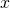, an addition chain foris an increasing sequence of positive integers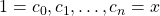such that for each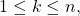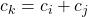for some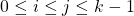. In 1937, Scholz conjectured that for each positive integer,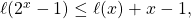where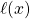denotes the minimal length of an addition chain for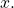In 1993, Aiello and Subbarao stated the apparently stronger conjecture that there is an addition chain for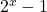with length equals to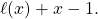We note that the Aiello–Subbarao conjecture is not stronger than the Scholz (also called the Scholz–Brauer) conjecture.

### Keywords

• Aiello–Subbarao’s conjecture
• Scholz–Brauer’s conjecture

• 11Y16
• 11Y55

### References

1. Aiello, W., & Subbarao, M. V. (1993). A conjecture in addition chains related to Scholz’s conjecture. Mathematics of Computation, 61(203), 17–23.
2. Bahig, H. M., & Nakamula, K. (2002). Some properties of nonstar steps in addition chains and new cases where the Scholz conjecture is true. Journal of Algorithms, 42(2), 304–316.
3. Bahig, H. M. (2006). Improved generation of minimal addition chains. Computing, 78, 161–172.
4. Bahig, H. M. (2011). Star reduction among minimal length addition chains. Computing, 91, 335–352.
5. Clift, N. M. (2011). Calculating optimal addition chains. Computing, 91, 265–284.
6. Flammenkamp, A. (1999). Integers with a small number of minimal addition chains. Discrete Mathematics, 205(1–3), 221–227.
7. Flammenkamp, A. (2020, June 7). Shortest addition chains. Universität Bielefeld personal webpage. http://wwwhomes.uni-bielefeld.de/achim/addition_chain.html
8. Gioia, A., Subbarao, M., & Sugunamma, M. (1962). The Scholz–Brauer problem in addition chains. Duke Mathematical Journal, 29, 481–487.
9. Knuth, D. E. (1997). The Art of Computer Programming: Seminumerical Algorithms (3 ed. Vol. 2, pp. 461–485). MA, Reading: Addison-Wesley.
10. Scholz, A. (1937). Jahresbericht. Jahresbericht der deutschen Mathematiker-Vereinigung, 47(2), 41–42.
11. Subbarao, M. (1989). Addition chains – some results and problems. In Mollin, R. A. (Ed.) Number Theory and Applications (pp. 555–574). Dordrecht: Kluwer Academic Publishers.
12. Thurber, E. G. (1973). The Scholz–Brauer problem on addition chains. Pacific Journal of Mathematics, 49(1), 229–242.
13. Utz, W. R. (1953). A note on the Scholz–Brauer problem on addition chains. Proceedings of the American Mathematical Society, 4, 462–463.